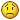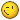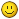# simple linux cam

• Reinhard
• Offline
• Platinum Member
•05 Apr 2022 03:08 #239298 by Reinhard
Replied by Reinhard on topic simple linux cam
What a f... (funny) editorThank you, but I would prefer and need your help with occ-math. Actually I coded formulas from brep specs like this:

First the line:
```gp_Pnt Util3D::calcLine(Handle(Geom_Line) l, double param) const {
const gp_Ax1& axis  = l->Position();
gp_Pnt        start(axis.Location().X(), axis.Location().Y(), axis.Location().Z());
gp_Dir        dir(axis.Direction().X(), axis.Direction().Y(), axis.Direction().Z());
gp_Pnt        ap = l->Value(param);
gp_Pnt        rv = start;

rv.SetX(start.X() + param * dir.X());
rv.SetY(start.Y() + param * dir.Y());
rv.SetZ(start.Z() + param * dir.Z());

l->D0(param, ap);

return rv;
}```

... and here the circle:
```gp_Pnt Util3D::calcCircle(Handle(Geom_Circle) c, double param) const {
const gp_Ax2& axis  = c->Position();
gp_Pnt        center(axis.Location().X(), axis.Location().Y(), axis.Location().Z());
gp_Dir        dir(axis.Direction());
gp_Dir        xDir(axis.XDirection());
gp_Dir        yDir(axis.YDirection());
double        cp = cos(param);
double        sp = sin(param);
gp_Pnt        ap = c->Value(param);
gp_Pnt        rv;

rv.SetX(center.X() + r * cp * xDir.X() + r * sp * yDir.X());
rv.SetY(center.Y() + r * cp * xDir.Y() + r * sp * yDir.Y());
rv.SetZ(center.Z() + r * cp * xDir.Z() + r * sp * yDir.Z());

c->D0(param, ap);

return rv;
}```
Both functions return the same result as D0 or Value from geom classes.
So I would appreciate it a lot if you could show me, how to calculate the "param" from given position.

• Reinhard
• Offline
• Platinum Member
•05 Apr 2022 04:38 #239300 by Reinhard
Replied by Reinhard on topic simple linux cam
Hi,

I just created a repo for this project .
Furthermore, I have created some clips , about what already works and where the journey should go.

µTube is not my friend, so I placed the clips in my repo. You'll have to download for watching.

Creating a clip is a good way of finding bugsThe following user(s) said Thank You: tommylight, Grotius

05 Apr 2022 11:13 - 05 Apr 2022 11:24 #239318 by Grotius
Replied by Grotius on topic simple linux cam
Hi Reinhard,

For retrieving the parameter i looked at the sketch lib of the Russian guy.
There was a lib called ELClib. This ELClib is huge, many functions.

```ElClib
//! Provides functions for basic geometric computations on
//! elementary curves such as conics and lines in 2D and 3D space.
//! This includes:
//! -   calculation of a point or derived vector on a 2D or
//! 3D curve where:
//! -   the curve is provided by the gp package, or
//! defined in reference form (as in the gp package),
//! and
//! -   the point is defined by a parameter,
//! -   evaluation of the parameter corresponding to a point
//! on a 2D or 3D curve from gp,
//! -   various elementary computations which allow you to
//! position parameterized values within the period of a curve.
//! Notes:
//! -   ElCLib stands for Elementary Curves Library.
//! -   If the curves provided by the gp package are not
//! explicitly parameterized, they still have an implicit
//! parameterization, analogous to that which they infer
//! for the equivalent Geom or Geom2d curves.```

For the line the function could look like:
```#include <Geom_Line.hxx>
#include <Geom_Circle.hxx>
#include <gp_Pnt.hxx>
#include <AIS_Shape.hxx>
#include <BRepBuilderAPI_MakeWire.hxx>
#include <BRepBuilderAPI_MakeEdge.hxx>
#include <ElCLib.hxx>

void OcctQtControl::on_pushButton_line_pressed()
{
//! The line example :
gp_Pnt a{0,0,0};
gp_Pnt b{100,100,0};
double linelenght=a.Distance(b);
std::cout<<"linelenght:"<<linelenght<<std::endl;

//! A non normalized line direction :
gp_Dir dir(b.X()-a.X(), b.Y()-a.Y(), b.Z()-a.Z());

//! Question is : how to calculate the "param" from given position.
//!
//! Input position : x50, y50, z50.
//! Output

gp_Pnt givenpos(50,50,0);
const gp_Ax1 Axis(a,dir);
double param=ElCLib::LineParameter(Axis,givenpos);

std::cout<<"param:"<<param<<std::endl;
}```

Output :
```linelenght:141.421
param:70.7107```
Last edit: 05 Apr 2022 11:24 by Grotius.

05 Apr 2022 11:49 #239322 by Grotius
Replied by Grotius on topic simple linux cam
```void function()
{
//! The arc example :
gp_Pnt start{0,0,0};
gp_Pnt end{100,100,0};
gp_Pnt center{50,0,0};

gp_Ax2 PlaneTop=gp::XOY();
PlaneTop.SetLocation(center);

gp_Pnt givenleftpos(0,0,0);
double param=ElCLib::CircleParameter(PlaneTop,givenleftpos);

gp_Pnt givenrightpos(100,0,0);
param=ElCLib::CircleParameter(PlaneTop,givenrightpos);
}```

Output :
```leftpos param in radians:3.14159

05 Apr 2022 11:55 #239323 by Grotius
Replied by Grotius on topic simple linux cam

• Reinhard
• Offline
• Platinum Member
•08 Apr 2022 04:02 #239617 by Reinhard
Replied by Reinhard on topic simple linux cam
Hi,

@Grotius
For retrieving the parameter i looked at the sketch lib of the Russian guy.
There was a lib called ELClib

Thank you for the keywordBest thing - "ELClib" is part of opencascadeI didn't have the time yet, to go for that. But its next on my todo list.

@all
I just finished the workflow for drill operations. I know - the most easy part.
Anyway - the steps from cad model to postprocessor output are there, so I can start with more challenging parts.

My 5axis test model - just the main drill operation for each side:

and resulting gcode file from Fanc-postprocessor (Fanuc because I know that dialect best):
```
( Job /media/Scratch/test2.ngc );
( generated by kutecam );
G40 G80;
T5;
M98 P100;
;
G90 A-20.000 B0.000 C0.000;
;
( Drill #0 - drill M8 core hole );
( T5 6,8mm Drill - D:6.6 L:125 );
N10 G0 G90 G54 X-22.500 Y-41.311 S1206 M3 T7;
G43 H5 Z50.000 M8;
G83 Z-15.000 R2.000 Q5 F96;
X22.420 Y-41.311;
X22.420 Y3.689;
X-22.500 Y3.689;
G0 G80 G90 Z150 M5;
M9;
M98 P100;
;
G90 A90.000 B0.000 C0.000;
;
( Drill #1 - drill 12.5 );
( T7 12,5mm Drill - D:12.5 L:150 );
N20 G0 G90 G54 X40.000 Y15.000 S637 M3 T5;
G43 H7 Z50.000 M8;
G83 Z53.000 R2.000 Q5 F100;
X40.000 Y95.000;
X-40.000 Y95.000;
X-40.000 Y15.000;
G0 G80 G90 Z150 M5;
M9;
;
G91 G28 Y0 Z0;
M30;
```

##### Attachments:
The following user(s) said Thank You: Grotius, silopolis

08 Apr 2022 21:06 #239699 by Grotius
Replied by Grotius on topic simple linux cam
Hi Reinhard,

So far looks good to me.
If you have any questions, no problem. If not too difficult i always try to provide info.

To inform.
I am trying to write a little c++ library.
The main goal is to provide some math functions for 3d.

Like finding intersection points. Trim objects etc for 3d geometry.

The idea came up when i was reverse engineering Draftsight with Ghidra and Ida past week.
I looked at the Api of Draftsight with Ghidra & Ida.
There are no secrets when opening a Elf file with these powerfull programs.

For now i came up with the example below, you can see a "Object" is created.
I like the idea that the Object is capable of intersecting different types on it's own. Like intersecting arc with line etc.

Also i came up with the idea of subclassing the gp_Pnt to gp_PntVec to create a array on the fly.
```OBJECT *lineA = new OBJECT();
lineA->setType(TYPE::line);
lineA->setPoints({{0,50,0},{100,50,0}});
occt_viewer->show_shape(lineA->getShape());

OBJECT *lineB = new OBJECT();
lineB->setType(TYPE::line);
lineB->setPoints({{50,0,0},{50,100,0}});
occt_viewer->show_shape(lineB->getShape());

gp_PntVec pvec;
lineA->getIntersections(*lineB,pvec);
pvec.push_back({100,1,1}); // To show the gp_Pnt vector usage.
pvec.print();

Output :
gp_PntVec at i:0 Value x: 50.000000 y: 50.000000 z: 0.000000
gp_PntVec at i:1 Value x: 100.000000 y: 1.000000 z: 1.000000```

• Reinhard
• Offline
• Platinum Member
•09 Apr 2022 03:29 #239705 by Reinhard
Replied by Reinhard on topic simple linux cam
Hi,

> So far looks good to me.

did you watch any of the videos?

> Like finding intersection points.

Something very strange is going on with opencascade boolean. The splitter only returns original wire, but no error nor warning and intersection creates cutpoints at locations, where no curve isSo I created a cutalgo for the workpiece. With some quick checks I sort the segments of the curve into 3 groups:
• completely inside the workpiece (rendered black)
• completely outside the workpiece (rendered white)
• possibly intersection (rendered cyan)
Now I have to cut the cyan segments only, using expensive maths.

It looks like "standard" maths leads to different result, than opencascade maths, so I have to dive deeper into that stuff##### Attachments:

• Reinhard
• Offline
• Platinum Member
•09 Apr 2022 04:56 #239706 by Reinhard
Replied by Reinhard on topic simple linux cam
Hi,

I don't know what to do anymore.
Here's the result of calculations. At first step I dedicated to cutting straight lines only (rendered in purple).
6 lines so 12 possible cutpoints. The cutpoints calculated are the little crosses at circle border.
To understand what's going on, I loaded a screenshot in incscape, where I extended the lines to cut.
4 lines seem to got the correct cutpoint, but only at the wrong side. The other cutpoints have no visual relation to any curve segment.

Does anybody have an idea, what I did wrong?

##### Attachments:

• Reinhard
• Offline
• Platinum Member
•09 Apr 2022 05:02 #239707 by Reinhard
Replied by Reinhard on topic simple linux cam
... and here's the code:
```Handle(Geom_Line) line = Handle(Geom_Line)::DownCast(c);
qDebug() << "cutCurve (line):" << first << " | " << last << " <> " << orientation;
double dx  = p1.X() - p0.X();
double dy  = p1.Y() - p0.Y();
double gf  = dy / dx;
double cf  = rMaster / sqrt(1 + gf * gf);
double dr  = sqrt(dx * dx + dy * dy);
double D   = p0.X() * p1.Y() - p1.X() * p0.Y();
double res = rMaster * rMaster * dr * dr - D * D;
double cx0 = cf * gf;
double cy0 = -cf;
double cx1 = -cx0;
double cy1 = -cy0;

if (res <= 0) qDebug() << "OUPS - fine calculations means no cutpoints?!?";
qDebug() << "cutpoints: " << cx0 << " / " << cy0
<<   "   and   " << cx1 << " / " << cy1;

Core().view3D()->createAxisCross({cx0, cy0, 0}, 2, nullptr, Quantity_NOC_BLACK);
Core().view3D()->createAxisCross({cx1, cy1, 0}, 2, nullptr, Quantity_NOC_WHITE);

double param0 = ElCLib::Parameter(line->Lin(), {cx0, cy0, p0.Z()});
double param1 = ElCLib::Parameter(line->Lin(), {cx1, cy1, p0.Z()});

if (param0 >= first && param0 <= last) {
qDebug() << "first cutpoint is part of line segment ==> "
<< first << " <> "  << param0 << " <> " << last;
//        edge = BRepBuilderAPI_MakeEdge(c, {cx0, cy0, p0.Z()}, p1);
}
else if (param1 >= first && param1 <= last) {
qDebug() << "second cutpoint is part of line segment ==> "
<< first << " <> "  << param1 << " <> " << last;
//        edge = BRepBuilderAPI_MakeEdge(c, p0, {cx1, cy1, p0.Z()});
}
else {
qDebug() << "?!?!? - none of the calculated cutpoints is inside of line parameters?!?"
<< first << " <> " << param0 << "|" << param1 << last;
edge = BRepBuilderAPI_MakeEdge(c, first, last);
}```